# Two Degree-of-Freedom PID Control for Setpoint Tracking

### Model Description

This example shows how to regulate the speed of an electric motor using two degrees-of-freedom PID control with set-point weighting. We use the PID Controller (2DOF) block in Simulink® as shown below.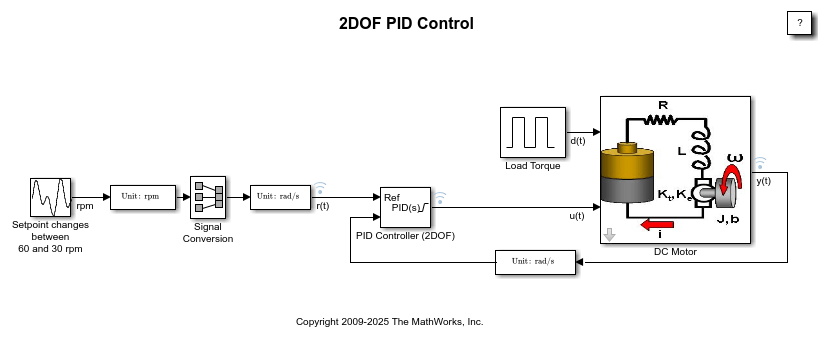Figure 1: Simulink model with two degree-of-freedom PID control of a DC motor.

To open this model, type `sldemo_pid2dof` in a MATLAB® terminal.

The electric motor is an armature-controlled DC motor. Voltage input controls the shaft speed of the motor. The block diagram of the motor is shown in Figure 2. The motor experiences a load torque(0-5 Nm).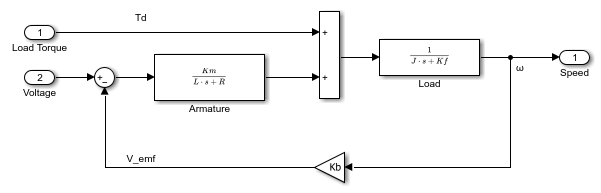Figure 2: Block diagram of the motor.

### Two Degree-of-Freedom PID Control

In contrast to the PID Controller block, the PID Controller (2DOF) block provides an extra degree of freedom to allow users to weight the setpoint as it passes through the proportional action channel and the derivative action channel. See the PID Controller (2DOF) help page or type `doc('PID Controller 2DOF')` in a MATLAB terminal for more details. The schematic of the PID Controller (2DOF) appearing in the model is shown below.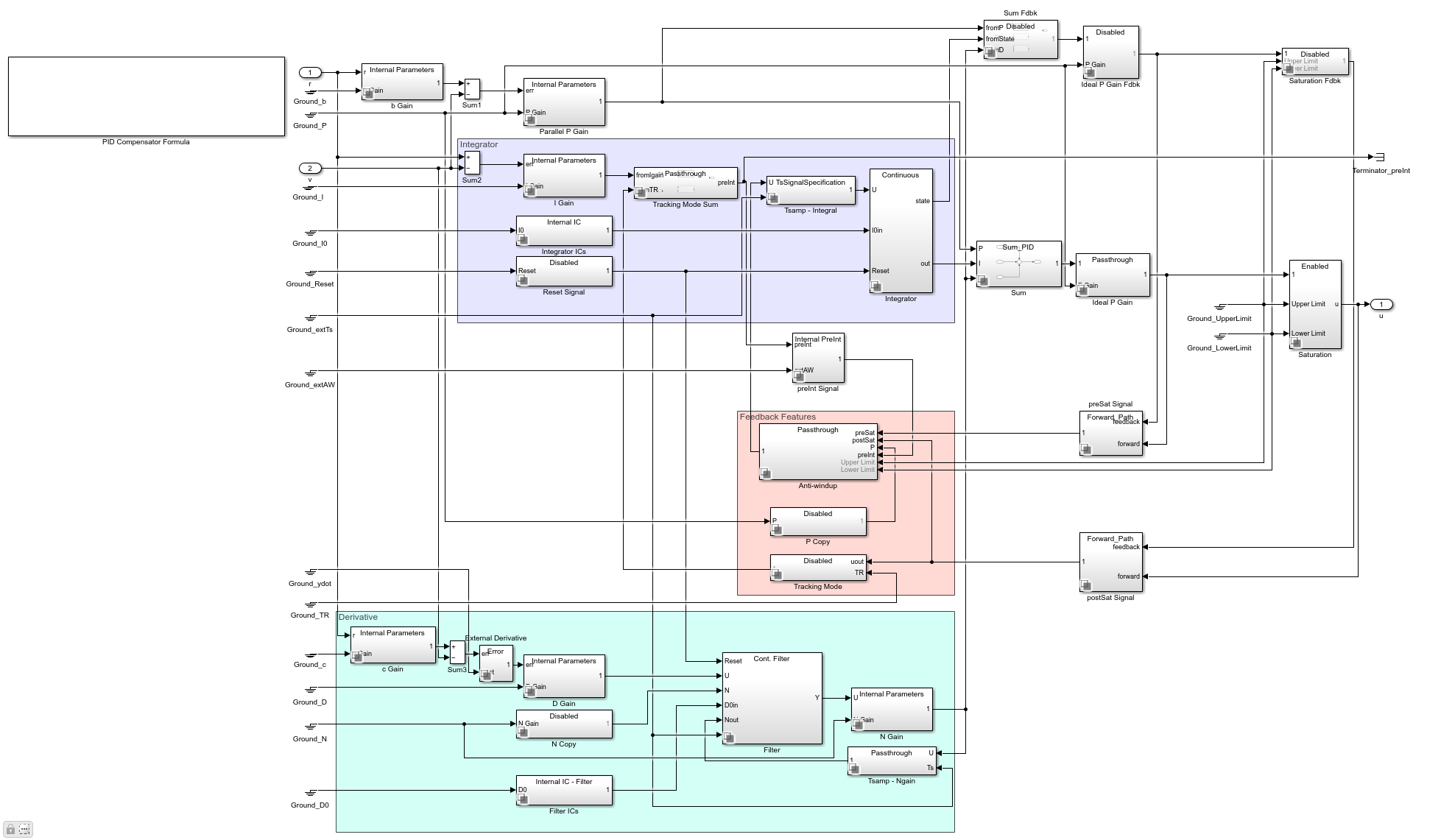Figure 3: Under-mask view of the PID Controller (2DOF).

As shown in Figure 3, the error signal seen by the proportional action is given by

``

The signal seen by the derivative action is

``

and the signal seen by the integral action is

``

In general, the setpoint weight c is chosen to be 0 to prevent undesirable transients upon a change in the setpoint, which is an effect known as derivative kick. The setpoint b affects the overshoot performance of the controller. Generally, a small b value reduces overshoot. However, smaller b values can also result in slower response to setpoint changes. For more details on picking the right setpoint values, see Reference .

Whenand, the behavior of the two degree-of-freedom PID controller is identical to a classical PID controller.

### Simulating with b = 1 and c = 1

Whenand, the behavior of the two degree-of-freedom PID controller is identical to a classical PID controller. The control signal, setpoint signal, and closed-loop response of the model are shown in Figure 4.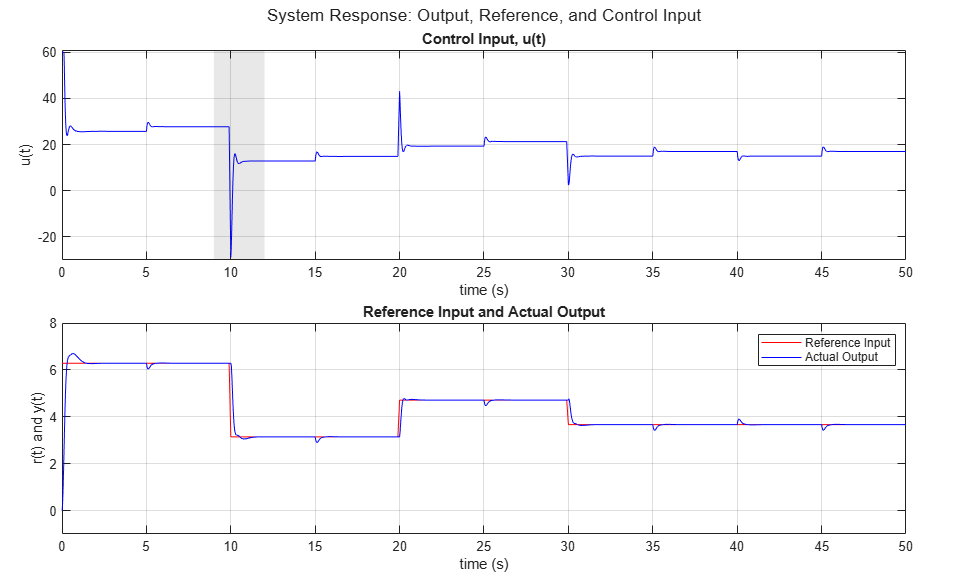Figure 4: Control signal, setpoint vs. measured output.

Figure 4 clearly shows the spikes in the control signal, which are caused by aggressive proportional and derivative response to the setpoint change. Modifying the b and c weights can make this response less aggressive, as shown next.

### Simulating with the b = 0 and c = 0

In this case, the two degree-of-freedom PID controller is known as I-PD where only the I action acts on the classical error signal, and the PD action acts only on the measured output.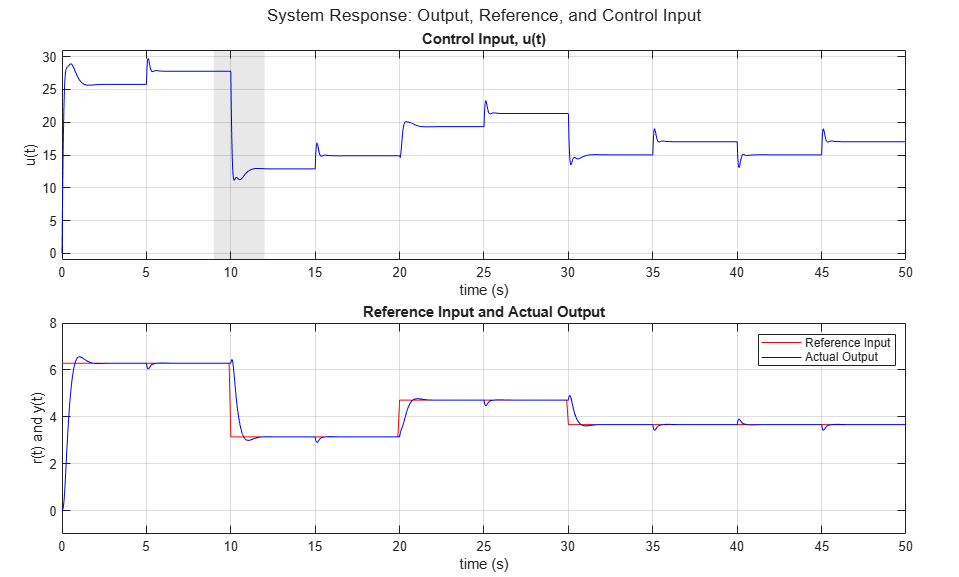Figure 5: Control signal, setpoint vs. measured output.

The simulation results clearly show the absence of large transients in the control signal due to the sudden changes in the setpoint.

See Reference  for more information on how b and c are chosen.

### Summary

The PID Controller (2DOF) block in Simulink supports two degree-of-freedom PID control. This block can be used for tracking complex setpoint profiles and moderating the impact of sudden setpoint changes on control signal transients. The PID tuner of Simulink® Control Design™ can be used to automatically tune all the gains (P, I, D, N, b, c) of the PID Controller (2DOF) block.

### References

1. K. Åström, T. Hägglund, Advanced PID Control, ISA, Research Triangle Park, NC, August 2005.